# dsp.FIRDecimator

Polyphase FIR decimator

## Description

The `dsp.FIRDecimator` System object™ resamples vector or matrix inputs along the first dimension. The FIR decimator (as shown in the schematic) conceptually consists of an anti-aliasing FIR filter followed by a downsampler.

The FIR filter filters the data in each channel of the input using a direct-form FIR filter. The FIR filter coefficients can be specified through the `Numerator` property, or can be automatically designed by the object using the `designMultirateFIR` function. The `designMultirateFIR` function designs an anti-aliasing FIR filter. The downsampler that follows the FIR filter downsamples each channel of filtered data by taking every M-th sample and discarding the M – 1 samples that follow. M is the value of the decimation factor that you specify. The resulting discrete-time signal has a sample rate that is 1/M times the original sample rate.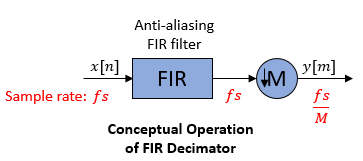Note that the actual object algorithm implements a direct-form FIR polyphase structure, an efficient equivalent of the combined system depicted in the diagram. For more details, see Algorithms.

To resample vector or matrix inputs along the first dimension:

1. Create the `dsp.FIRDecimator` object and set its properties.

2. Call the object with arguments, as if it were a function.

Under specific conditions, this System object also supports SIMD code generation. For details, see Code Generation.

## Creation

### Syntax

``firdecim = dsp.FIRDecimator``
``firdecim = dsp.FIRDecimator(M)``
``firdecim = dsp.FIRDecimator(M,'Auto')``
``firdecim = dsp.FIRDecimator(M,num)``
``firdecim = dsp.FIRDecimator(___,Name,Value)``
``firdecim = dsp.FIRDecimator(M,'legacy')``

### Description

example

````firdecim = dsp.FIRDecimator` returns an FIR decimator object with a decimation factor of 2. The object designs the FIR filter coefficients using the `designMultirateFIR(1,2)` function.```
````firdecim = dsp.FIRDecimator(M)` returns an FIR decimator with the integer-valued `DecimationFactor` property set to `M`. The object designs its filter coefficients based on the decimation factor `M` that you specify while creating the object, using the `designMultirateFIR(1,M)` function. The designed filter corresponds to a lowpass with a cutoff at π/`M` in radial frequency units.```
````firdecim = dsp.FIRDecimator(M,'Auto')` returns an FIR decimator with the `NumeratorSource` property set to `'Auto'`. In this mode, every time there is an update in the decimation factor, the object redesigns the filter using `designMultirateFIR(1,M)`.```
````firdecim = dsp.FIRDecimator(M,num)` returns an FIR decimator with the `DecimationFactor` property set to `M` and the `Numerator` property set to `num`.```
````firdecim = dsp.FIRDecimator(___,Name,Value)` returns an FIR decimator object with each specified property set to the specified value. Enclose each property name in quotes. You can use this syntax with any previous input argument combinations.```
````firdecim = dsp.FIRDecimator(M,'legacy')` returns an FIR decimator where the filter coefficients are designed using `fir1(35,0.4)`. The designed filter has a cutoff frequency of 0.4π radians/sample.```

## Properties

expand all

Unless otherwise indicated, properties are nontunable, which means you cannot change their values after calling the object. Objects lock when you call them, and the `release` function unlocks them.

If a property is tunable, you can change its value at any time.

### Main Properties

Decimation factor M, specified as a positive integer. The FIR decimator reduces the sampling rate of the input by this factor. The number of input rows must be a multiple of the decimation factor.

Data Types: `single` | `double` | `int8` | `int16` | `int32` | `int64` | `uint8` | `uint16` | `uint32` | `uint64`

FIR filter coefficient source, specified as one of the following:

• `'Property'` –– The numerator coefficients are specified through the `Numerator` property.

• `'Input port'` –– The numerator coefficients are specified as an input to the object algorithm.

• `'Auto'` –– The numerator coefficients are designed automatically using the `designMultirateFIR(1,M)` function.

Numerator coefficients of the FIR filter, specified as a row vector in powers of z–1. The following equation defines the system function for a filter of length N+1:

`$H\left(z\right)=\sum _{l=0}^{N}{b}_{l}{z}^{-l}$`

The vector b = [b0, b1, …, bN] represents the vector of filter coefficients.

To prevent aliasing as a result of downsampling, the filter transfer function should have a normalized cutoff frequency no greater than 1/`M`. To design an effective anti-aliasing filter, use the `designMultirateFIR` function. For an example, see Decimate Sum of Sine Waves.

#### Dependencies

This property is visible only when you set `NumeratorSource` to `'Property'`.

When `NumeratorSource` is set to `'Auto'`, the numerator coefficients are automatically redesigned using `designMultirateFIR(1,M)`. To access the filter coefficients in the automatic design mode, type `objName.``Numerator` in the MATLAB® command prompt.

Data Types: `single` | `double` | `int8` | `int16` | `int32` | `int64` | `uint8` | `uint16` | `uint32` | `uint64`
Complex Number Support: Yes

Specify the implementation of the FIR filter as either ```Direct form``` or `Direct form transposed`.

### Code Generation Properties

Allow arbitrary frame length for fixed-size input signals in the generated code, specified as `true` or `false`. When you specify:

• `true` –– The input frame length does not have to be a multiple of the decimation factor. The output of the object in the generated code is a variable-size array.

• `false` –– The input frame length must be a multiple of the decimation factor.

When you specify variable-size signals, the input frame length can be arbitrary and the object ignores this property in the generated code. When you run this object in MATLAB, the object supports arbitrary input frame lengths for fixed-size and variable-size signals and this property does not affect the object behavior.

Data Types: `logical`

### Fixed-Point Properties

Flag to use full-precision rules for fixed-point arithmetic, specified as one of the following:

• `true` –– The object computes all internal arithmetic and output data types using the full-precision rules. These rules provide the most accurate fixed-point numerics. In this mode, other fixed-point properties do not apply. No quantization occurs within the object. Bits are added, as needed, to ensure that no roundoff or overflow occurs.

• `false` –– Fixed-point data types are controlled through individual fixed-point property settings.

For more information, see Full Precision for Fixed-Point System Objects and Set System Object Fixed-Point Properties.

Rounding method for fixed-point operations. For more details, see rounding mode.

#### Dependencies

This property is not visible and has no effect on the numerical results when the following conditions are met:

• `FullPrecisionOverride` set to `true`.

• `FullPrecisionOverride` set to `false`, `ProductDataType` set to `'Full precision'`, `AccumulatorDataType` set to ```'Full precision'```, and `OutputDataType` set to `'Same as accumulator'`.

Under these conditions, the object operates in full precision mode.

Overflow action for fixed-point operations, specified as one of the following:

• `'Wrap'` –– The object wraps the result of its fixed-point operations.

• `'Saturate'` –– The object saturates the result of its fixed-point operations.

For more details on overflow actions, see overflow mode for fixed-point operations.

#### Dependencies

This property is not visible and has no effect on the numerical results when the following conditions are met:

• `FullPrecisionOverride` set to `true`.

• `FullPrecisionOverride` set to `false`, `OutputDataType` set to `'Same as accumulator'`, `ProductDataType` set to ```'Full precision'```, and `AccumulatorDataType` set to `'Full precision'`

Under these conditions, the object operates in full precision mode.

Data type of the FIR filter coefficients, specified as:

• `Same word length as input` –– The word length of the coefficients is the same as that of the input. The fraction length is computed to give the best possible precision.

• `Custom` –– The coefficients data type is specified as a custom numeric type through the `CustomCoefficientsDataType` property.

Word and fraction lengths of the coefficients data type, specified as an autosigned `numerictype` (Fixed-Point Designer) with a word length of 16 and a fraction length of 15.

#### Dependencies

This property applies when you set the `CoefficientsDataType` property to `Custom`.

Data type of the product output in this object, specified as one of the following:

• `'Full precision'` –– The product output data type has full precision.

• `'Same as input'` –– The object specifies the product output data type to be the same as that of the input data type.

• `'Custom'` –– The product output data type is specified as a custom numeric type through the `CustomProductDataType` property.

For more information on the product output data type, see Multiplication Data Types.

#### Dependencies

This property applies when you set `FullPrecisionOverride` to `false`.

Word and fraction lengths of the product data type, specified as an autosigned numeric type with a word length of 32 and a fraction length of 30.

#### Dependencies

This property applies only when you set `FullPrecisionOverride` to `false` and `ProductDataType` to `'Custom'`.

Data type of an accumulation operation in this object, specified as one of the following:

• `'Full precision'` –– The accumulation operation has full precision.

• `'Same as product'` –– The object specifies the accumulator data type to be the same as that of the product output data type.

• `'Same as input'` –– The object specifies the accumulator data type to be the same as that of the input data type.

• `'Custom'` –– The accumulator data type is specified as a custom numeric type through the `CustomAccumulatorDataType` property.

#### Dependencies

This property applies when you set `FullPrecisionOverride` to `false`.

Word and fraction lengths of the accumulator data type, specified as an autosigned numeric type with a word length of 32 and a fraction length of 30.

#### Dependencies

This property applies only when you set `FullPrecisionOverride` to `false` and `AccumulatorDataType` to `'Custom'`.

Data type of the object output, specified as one of the following:

• `'Same as accumulator'` –– The output data type is the same as that of the accumulator output data type.

• `'Same as input'` –– The output data type is the same as that of the input data type.

• `'Same as product'` –– The output data type is the same as that of the product output data type.

• `'Custom'` –– The output data type is specified as a custom numeric type through the `CustomOutputDataType` property.

#### Dependencies

This property applies when you set `FullPrecisionOverride` to `false`.

Word and fraction lengths of the output data type, specified as an autosigned numeric type with a word length of 16 and a fraction length of 15.

#### Dependencies

This property applies only when you set `FullPrecisionOverride` to `false` and `OutputDataType` to `'Custom'`.

## Usage

### Syntax

``y = firdecim(x)``
``y = firdecim(x,num)``

### Description

example

````y = firdecim(x)` outputs the filtered and downsampled values, `y`, of the input signal, `x`.```
````y = firdecim(x,num)` uses the FIR filter, `num`, to decimate the input signal. This configuration is valid only when the `'NumeratorSource'` property is set to `'Input port'`.```

### Input Arguments

expand all

Data input, specified as a column vector or a matrix of size P-by-Q. The columns in the input signal represent Q independent channels.

Under most conditions, the number of inputs rows P can be arbitrary and does not have to be a multiple of the `DecimationFactor` property. See this table for details.

Input SignalWhen you Run Object in MATLABWhen you Generate Code Using MATLAB Coder™
Fixed-sizeObject supports arbitrary input frame lengthObject supports arbitrary input frame length when you set `AllowArbitraryInputLength` to `true` while generating code
Variable-sizeObject supports arbitrary input frame lengthObject supports arbitrary input frame length

Variable-size signals change in frame length once you lock the object while the fixed-size signals remain constant. When the object does not support arbitrary frame length, the input frame length must be a multiple of the `DecimationFactor` property.

This object does not support complex unsigned fixed-point inputs.

Data Types: `single` | `double` | `int8` | `int16` | `int32` | `int64` | `uint8` | `uint16` | `uint32` | `uint64` | `fi`
Complex Number Support: Yes

FIR filter coefficients, specified as a row vector.

#### Dependencies

This input is accepted only when the `'NumeratorSource'` property is set to `'Input port'`.

Data Types: `single` | `double` | `int8` | `int16` | `int32` | `int64` | `uint8` | `uint16` | `uint32` | `uint64` | `fi`
Complex Number Support: Yes

### Output Arguments

expand all

FIR decimator output, returned as a column vector or a matrix. When the input is of size P-by-Q, and P is not a multiple of the decimation factor M, the output signal has an upper bound size of `ceil`(P/M)-by-Q. If P is a multiple of the decimation factor, then the output is of size (P/M)-by-Q.

Data Types: `single` | `double` | `int8` | `int16` | `int32` | `int64` | `uint8` | `uint16` | `uint32` | `uint64` | `fi`
Complex Number Support: Yes

## Object Functions

To use an object function, specify the System object as the first input argument. For example, to release system resources of a System object named `obj`, use this syntax:

`release(obj)`

expand all

 `freqz` Frequency response of discrete-time filter System object `fvtool` Visualize frequency response of DSP filters `info` Information about filter System object `cost` Estimate cost of implementing filter System object `polyphase` Polyphase decomposition of multirate filter `generatehdl` Generate HDL code for quantized DSP filter (requires Filter Design HDL Coder) `impz` Impulse response of discrete-time filter System object `coeffs` Returns the filter System object coefficients in a structure
 `step` Run System object algorithm `release` Release resources and allow changes to System object property values and input characteristics `reset` Reset internal states of System object

## Examples

collapse all

Decimate a sum of sine waves by a factor of 2 and by a factor of 4.

Start with a cosine wave that has an angular frequency of $\frac{\pi }{4}$ radians/sample.

`x = cos(pi/4*(0:95)');`

Design Default Filter

Create a `dsp.FIRDecimator` object. To prevent aliasing, the object uses an anti-aliasing lowpass filter before downsampling. By default, the anti-aliasing lowpass filter is designed using the `designMultirateFIR` function. The function designs the filter based on the decimation factor that you specify, and stores the coefficients in the `Numerator` property. For a decimation factor of 2, the object designs the coefficients using `designMultirateFIR(1,2)`.

`firdecim = dsp.FIRDecimator(2)`
```firdecim = dsp.FIRDecimator with properties: Main DecimationFactor: 2 NumeratorSource: 'Property' Numerator: [0 -1.0054e-04 0 3.8704e-04 0 -0.0010 0 0.0022 0 ... ] Structure: 'Direct form' Show all properties ```

Visualize the filter response using `fvtool`. The designed filter meets the ideal filter constraints that are marked in red. The cutoff frequency is approximately half the spectrum.

`fvtool(firdecim)`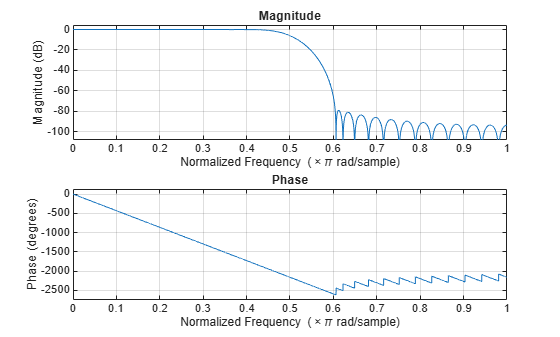Decimate by 2

Decimate the cosine signal by a factor of 2.

`y = firdecim(x);`

Plot the original and the decimated signals. In order to plot the two signals on the same plot, you must account for the output delay of the FIR decimator and the scaling introduced by the filter. Use the `outputDelay` function to compute the `delay` value introduced by the decimator. Shift the output by this delay value.

Visualize the input and the resampled signals. After a short transition, the output converges to a cosine of frequency $\frac{\pi }{2}$ as expected, which is twice the frequency of the input signal $\frac{\pi }{4}$. Due to the decimation factor of 2, the output samples coincide with every other input sample.

```[delay,FsOut] = outputDelay(firdecim); nx = (0:length(x)-1); ty = (0:length(y)-1)/FsOut-delay; stem(ty,y,'filled',MarkerSize=4); hold on; stem(nx,x); hold off; xlim([-10,22]) ylim([-2.5 2.5]) legend('Decimated by 2 (y)','Input signal (x)');```Add a High Frequency Component to Input and Decimate

Add another frequency component to the input signal, a sine with an angular frequency of $\frac{2\pi }{3}$ radians/sample. Since $\omega =\text{\hspace{0.17em}}\frac{2\pi }{3}$ is above the FIR lowpass cutoff, $\frac{\pi }{2}$, the frequency $\frac{2\pi }{3}$ radians/sample is filtered out from the signal.

```xhigh = x + 0.2*sin(2*pi/3*(0:95)'); release(firdecim) yhigh = firdecim(xhigh);```

Plot the input signal, decimated signal, and the output of the low frequency component. The decimated signal `yhigh `has the high frequency component filtered out. `yhigh` is almost identical to the output of the low frequency component `y`.

```stem(ty,yhigh,'filled',MarkerSize=4); hold on; stem(nx,xhigh); stem(ty,y,':m',MarkerSize=7); hold off; xlim([-10,22]) ylim([-2.5 2.5]) legend('Decimated by 2 (yhigh)',... 'Input signal with the high tone added (xhigh)',... 'Decimated by 2 - low tone only (y)');```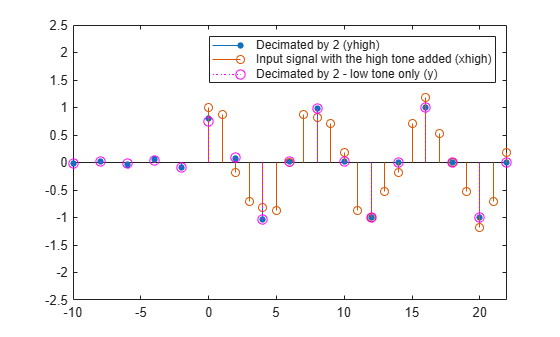Decimate by 4 in Automatic Filter Design Mode

Now decimate by a factor of 4. In order for the filter design to be updated automatically based on the new decimation factor, set the `NumeratorSource` property to `'Auto'`. Alternately, you can pass `'Auto'` as the keyword while creating the object. The object then operates in the automatic filter design mode. Every time there is a change in the decimation factor, the object updates the filter design.

```release(firdecim) firdecim.NumeratorSource = 'Auto'; firdecim.DecimationFactor = 4```
```firdecim = dsp.FIRDecimator with properties: Main DecimationFactor: 4 NumeratorSource: 'Auto' Structure: 'Direct form' Show all properties ```

To access the filter coefficients in the automatic mode, type `firdecim.Numerator` in the MATLAB command prompt.

The designed filter occupies a narrower passband that is approximately a quarter of the spectrum.

`fvtool(firdecim)`Decimate the cosine signal by a factor of 4. After a short transition, the output converges to a cosine of frequency $\pi$ as expected, which is four times the lower frequency component of the input signal $\frac{\pi }{4}$. This time, the amplitude of the output is half the amplitude of the input since the gain of the FIR at $\omega =\frac{\pi }{4}$ is exactly $\frac{1}{2}$. The high frequency component $\frac{2\pi }{3}$ diminishes by the lowpass FIR whose cutoff frequency is $\frac{\pi }{4}$.

`yAuto = firdecim(xhigh);`

Plot the input signal with the high frequency component added, low frequency component scaled by 1/2, and the decimated signal. Recalculate the output delay and the output sample rate since the decimation factor has changed.

```[delay,FsOut] = outputDelay(firdecim); tyAuto = (0:length(yAuto)-1)/FsOut-delay; stem(tyAuto,yAuto,'filled',MarkerSize=4); hold on; stem(nx,xhigh); stem(nx,x/2,'m:',MarkerSize=7); hold off; xlim([-20,36]) ylim([-2.5 2.5]) legend('Decimated by 4 (yAuto)',... 'Input signal with the high frequency component added (xhigh)',... 'Low tone input scaled by 1/2');```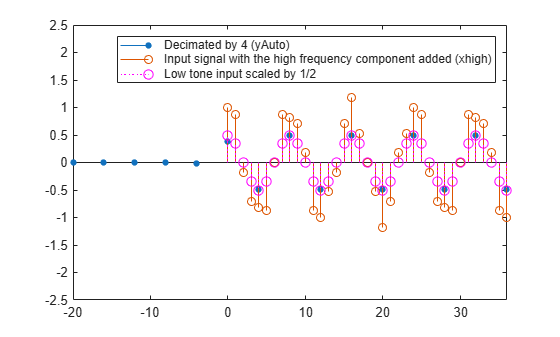Reduce the sample rate of an audio signal by a factor of 2 and play the decimated signal using the `audioDeviceWriter` object.

Note: If you are using R2016a or an earlier release, replace each call to the object with the equivalent `step `syntax. For example, `obj(x) `becomes `step(obj,x)`.

Note: The `audioDeviceWriter` System object™ is not supported in MATLAB Online.

Create a `dsp.AudioFileReader` object. The default audio file read by the object has a sample rate of 22050 Hz.

```afr = dsp.AudioFileReader('OutputDataType',... 'single');```

Create a `dsp.FIRDecimator` object and specify the decimation factor to be 2. The object designs the filter using `designMultirateFIR(1,2)` and stores the coefficients in the `Numerator` property of the object.

`firdecim = dsp.FIRDecimator(2)`
```firdecim = dsp.FIRDecimator with properties: Main DecimationFactor: 2 NumeratorSource: 'Property' Numerator: [0 -1.0054e-04 0 3.8704e-04 0 -0.0010 0 0.0022 0 ... ] Structure: 'Direct form' Show all properties ```

Create an `audioDeviceWriter` object. Specify the sample rate to be 22050/2.

`adw = audioDeviceWriter(22050/2)`
```adw = audioDeviceWriter with properties: Device: 'Default' SampleRate: 11025 Show all properties ```

Read the audio signal using the file reader object, decimate the signal by a factor of 2, and play the decimated signal.

```while ~isDone(afr) frame = afr(); y = firdecim(frame); adw(y); end release(afr); pause(0.5); release(adw);```

expand all

## Algorithms

The FIR decimation filter is implemented efficiently using a polyphase structure. For more details on polyphase filters, see Polyphase Subfilters.

To derive the polyphase structure, start with the transfer function of the FIR filter:

`$H\left(z\right)={b}_{0}+{b}_{1}{z}^{-1}+...+{b}_{N}{z}^{-N}$`

N+1 is the length of the FIR filter.

You can rearrange this equation as follows:

`$H\left(z\right)=\begin{array}{c}\left({b}_{0}+{b}_{M}{z}^{-M}+{b}_{2M}{z}^{-2M}+..+{b}_{N-M+1}{z}^{-\left(N-M+1\right)}\right)+\\ {z}^{-1}\left({b}_{1}+{b}_{M+1}{z}^{-M}+{b}_{2M+1}{z}^{-2M}+..+{b}_{N-M+2}{z}^{-\left(N-M+1\right)}\right)+\\ \begin{array}{c}⋮\\ {z}^{-\left(M-1\right)}\left({b}_{M-1}+{b}_{2M-1}{z}^{-M}+{b}_{3M-1}{z}^{-2M}+..+{b}_{N}{z}^{-\left(N-M+1\right)}\right)\end{array}\end{array}$`

M is the number of polyphase components, and its value equals the decimation factor that you specify.

You can write this equation as:

`$H\left(z\right)={E}_{0}\left({z}^{M}\right)+{z}^{-1}{E}_{1}\left({z}^{M}\right)+...+{z}^{-\left(M-1\right)}{E}_{M-1}\left({z}^{M}\right)$`

E0(zM), E1(zM), ..., EM-1(zM) are the polyphase components of the FIR filter H(z).

Conceptually, the FIR decimation filter contains a lowpass FIR filter followed by a downsampler.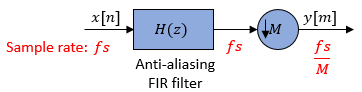Replace H(z) with its polyphase representation.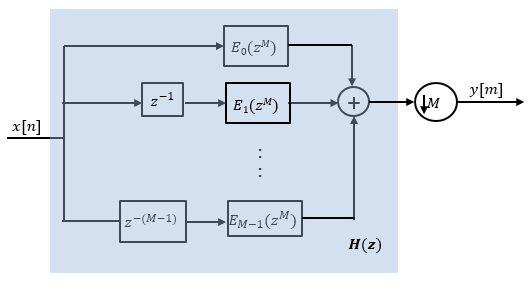Here is the multirate noble identity for decimation.Applying the noble identity for decimation moves the downsampling operation to before the filtering operation. This move enables you to filter the signal at a lower rate.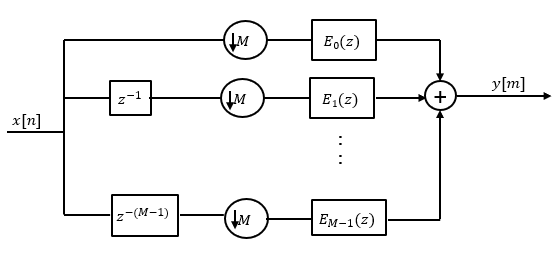You can replace the delays and the decimation factor at the input with a commutator switch. The switch starts on the first branch 0 and moves in the counterclockwise direction as shown in this diagram. The accumulator at the output receives the processed input samples from each branch of the polyphase structure and accumulates these processed samples until the switch goes to branch 0. When the switch goes to branch 0, the accumulator outputs the accumulated value.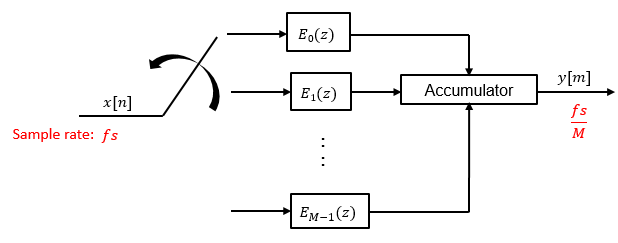When the first input sample is delivered, the switch feeds this input to the branch 0 and the decimator computes the first output value. As more input samples come in, the switch moves in the counter clockwise direction through branches M−1, M−2, and all the way up to branch 0, delivering one sample at a time to each branch. When the switch comes to branch 0, the decimator outputs the next set of output values. This process continues as data keeps coming in. Every time the switch comes to the branch 0, the decimator outputs y[m]. The decimator effectively outputs one sample for every M samples it receives. Hence the sample rate at the output of the FIR decimation filter is fs/M.

## Version History

Introduced in R2012a

expand all